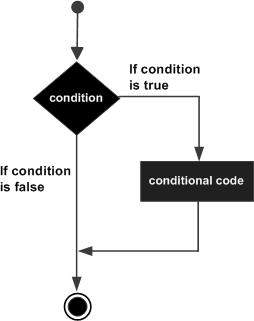# PL/SQL - IF-THEN Statement

It is the simplest form of the IF control statement, frequently used in decision-making and changing the control flow of the program execution.

The IF statement associates a condition with a sequence of statements enclosed by the keywords THEN and END IF. If the condition is TRUE, the statements get executed, and if the condition is FALSE or NULL, then the IF statement does nothing.

## Syntax

Syntax for IF-THEN statement is −

```IF condition THEN
S;
END IF;
```

Where condition is a Boolean or relational condition and S is a simple or compound statement. Following is an example of the IF-THEN statement −

```IF (a <= 20) THEN
c:= c+1;
END IF;
```

If the Boolean expression condition evaluates to true, then the block of code inside the if statement will be executed. If the Boolean expression evaluates to false, then the first set of code after the end of the if statement (after the closing end if) will be executed.

## Flow Diagram## Example 1

Let us try an example that will help you understand the concept −

```DECLARE
a number(2) := 10;
BEGIN
a:= 10;
-- check the boolean condition using if statement
IF( a < 20 ) THEN
-- if condition is true then print the following
dbms_output.put_line('a is less than 20 ' );
END IF;
dbms_output.put_line('value of a is : ' || a);
END;
/
```

When the above code is executed at the SQL prompt, it produces the following result −

```a is less than 20
value of a is : 10

PL/SQL procedure successfully completed.
```

## Example 2

Consider we have a table and few records in the table as we had created in PL/SQL Variable Types

```DECLARE
c_id customers.id%type := 1;
c_sal  customers.salary%type;
BEGIN
SELECT  salary
INTO  c_sal
FROM customers
WHERE id = c_id;
IF (c_sal <= 2000) THEN
UPDATE customers
SET salary =  salary + 1000
WHERE id = c_id;
dbms_output.put_line ('Salary updated');
END IF;
END;
/
```

When the above code is executed at the SQL prompt, it produces the following result −

```Salary updated

PL/SQL procedure successfully completed.
```
plsql_conditional_control.htm